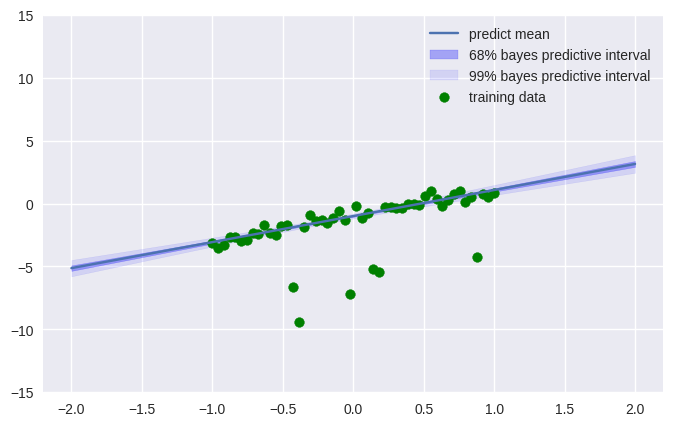# 【Jax, Numpyro】Regression Model practice

## はじめに

import jax.numpy as np
from jax import random

import matplotlib.pyplot as plt
import numpy as onp
import seaborn as sns

import numpyro
from numpyro.infer import MCMC, NUTS, Predictive
from numpyro import plate, sample, handlers
import numpyro.distributions as dist

plt.style.use("seaborn")


## Simple regression

Simple regression model $y = ax + b + \epsilon$ is built with likelihood function $L(a, b, \sigma )$ and prior distribution $p(a, b, \sigma)$. Now, we choice

\begin{align} L(a, b, \sigma ) &= \prod _ i {\rm Normal}(y _ i \mid ax _ i + b, \sigma) \\ p(a, b, \sigma) &= {\rm Normal}(a\mid 0, 100){\rm Normal}(b\mid 0, 100){\rm LogNormal}(a\mid 0, 10) \end{align}

This assume that observed data $(x _ i, y _ i)$ is i.i.d and $a, b, c$ are independet each other and this model represent below generative flow.

\begin{align} a &\sim {\rm Normal}(0, 100) \\ b &\sim {\rm Normal}(0, 100) \\ \sigma & \sim {\rm LogNormal}(0, 10) \\ y _ i &\sim {\rm Normal}(ax _ i+b, \sigma) \end{align}

In NumPyro coding, we can focus on writing generative flow, and which random variable are (conditional) independent.

def model(x, y, N=100):
a = sample("a", dist.Normal(0, 100))
b = sample("b", dist.Normal(0, 100))

sigma = sample("sigma", dist.LogNormal(0, 10))

with plate("data", N):
sample("obs", dist.Normal(a *x + b, sigma), obs=y)


### prior sampling

Now, we get samples from prior distribution using above generative flow model(x, y, N). That prior sampling is not often good from point of view fitting to data because this model have no information of data.

prior_model_trace = handlers.trace(handlers.seed(model, random.PRNGKey(0)))

x = np.linspace(-1, 1, 1000)
prior_model_exec = prior_model_trace.get_trace(x=x,
y=None,
N=1000)
y = prior_model_exec["obs"]["value"]
print(y.shape)
plt.plot(x, y, "o")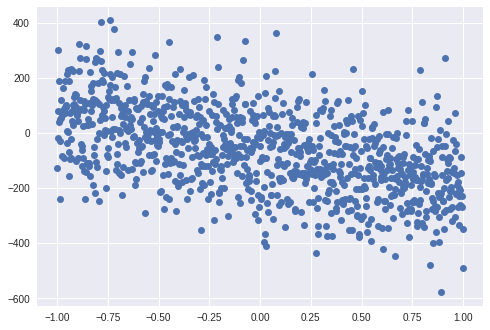### toy data

Toy data come from below.

\begin{align} y &= ax +b + \epsilon\\ \epsilon &\sim {\rm Normal}(0, 0.3) \end{align}

where $a$ and $b$ are set to -2 and 3 in this turorial.

def toy_data(a, b, N):
x = np.linspace(-1, 1, N)
y = a * x + b + 0.3*random.normal(random.PRNGKey(1), x.shape)
return x, y

x_data, y_data = toy_data(-2, 3, 50)
plt.plot(x_data, y_data, "o")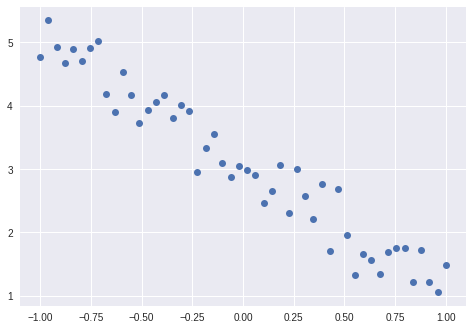### inference by NUTS

A posterior distribution is represented as below.

$$p(a, b, c \mid X, Y) = \frac {p(X, Y \mid a, b, \sigma)p(a, b, \sigma)} {\int _ {a, b, \sigma}p(X, Y \mid a, b, \sigma)p(a, b, \sigma){\rm d}a{\rm d}b{\rm d}\sigma}$$

where $X, Y$ are dataset ${x _ 1, ..., x _ N}$ and ${y _ 1, ..., y _ N}$ which have already got. This distribution is often difficult to earn closed form. Therefore, now, let's use NUTS(No U Turn Sampler) which is kind of MCMC.

kernel = NUTS(model)
mcmc = MCMC(kernel, num_warmup=300, num_samples=1000)


NumPyro's MCMC automatically handles random variable which is constrain. We don't need bijectors $f$ which map constraint space to real space (When using TensorFlow Probability, we need set bijectors fitted to each random variables).

mcmc.run(random.PRNGKey(1), x=x_data, y=y_data, N=x_data.shape)


In google colab, calculation was finished in 8 seconds.

mcmc.print_summary()

mean       std    median      5.0%     95.0%     n_eff     r_hat
a     -2.01      0.07     -2.01     -2.13     -1.90    942.24      1.00
b      3.07      0.04      3.07      3.00      3.15    932.36      1.00
sigma      0.31      0.03      0.31      0.26      0.36   1188.74      1.00

Number of divergences: 0


### result

samples = mcmc.get_samples()
a_samples = samples["a"].squeeze()
b_samples = samples["b"].squeeze()
sigma_samples = samples["sigma"].squeeze()

plt.figure(figsize=(12, 4))
plt.subplot(121)
sns.distplot(a_samples, bins=20)
plt.subplot(122)
plt.plot(a_samples)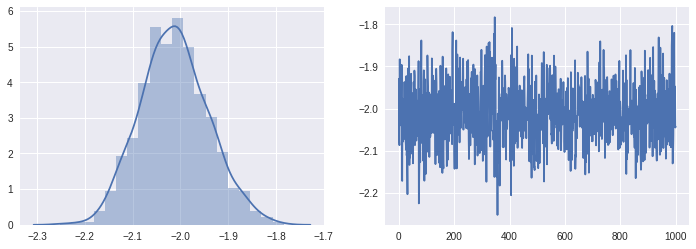plt.figure(figsize=(12, 4))
plt.subplot(121)
sns.distplot(b_samples, bins=20)
plt.subplot(122)
plt.plot(b_samples)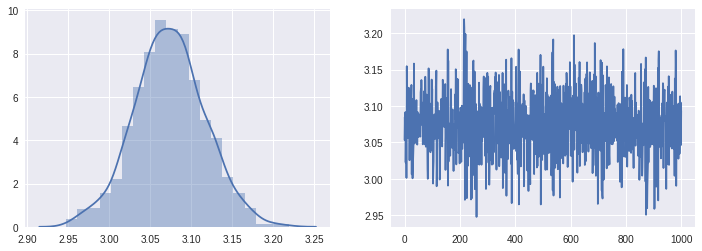plt.figure(figsize=(12, 4))
plt.subplot(121)
sns.distplot(sigma_samples, bins=20)
plt.subplot(122)
plt.plot(sigma_samples)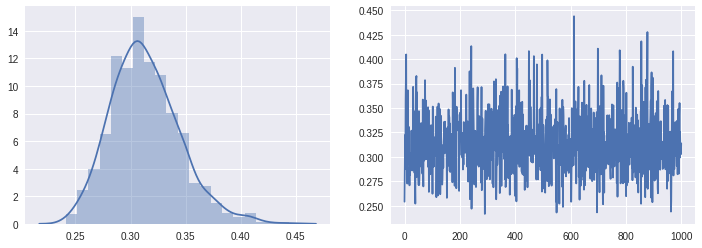### predictive distribution

A predictive distribution is represented as below equation called bayes predictive distribution.

$$p ^ *(y \mid x) = \int _ {a, b, \sigma} p(y\mid x, a, b, \sigma)p(a, b, \sigma \mid X, Y){\rm d}a{\rm d}b{\rm d}\sigma$$

where $X, Y$ are data which we have got already. $p(a,b, \sigma \mid X, Y)$ is called posterior distribution. Posterior distirbution had got as samples by above code instead of concreate mathmetical closed form.

We can get predictive distribution with below flow.

\begin{align} {\rm for\ \ k=1:K}&& \\ a _ k, b _ k, \sigma _ k &\sim p(a, b, \sigma \mid X, Y) \end{align}

then,

\begin{align} {\rm for\ \ i=1:N}&{\rm, \ \ \ k = 1: K} \\ y _ i &\sim p(y _ i\mid x _ i, a _ k, b _ k, \sigma _ k) \end{align}

The first sampling have already done with MCMC (now, $K = 1000$). All we need is sampling from $p(y _ i \mid x _ i , a _ k , b _ k , \sigma _ k)$ for each data points $x _ i$ using $K$ samples of parameters.

This $K \times N$ loops is so heavy for python that late enough for sunset. Fortunately, jax have great function vmap and jit to accelerate calcuration. This function little difficult for first user, so NumPyro have a magic class numpyro.infer.Predictive to built bayes predictive distribution.

predictive = Predictive(model, samples)


OK. Let's get predictive distribution as samples.

index_points = np.linspace(-2., 2., 1500)

predictive_samples = predictive.get_samples(
random.PRNGKey(1),
index_points,
None,
index_points.shape)["obs"]

mean = predictive_samples.mean(0)
std = predictive_samples.std(0)

lower1 = mean - std
upper1 = mean + std
lower3 = mean - 3*std
upper3 = mean + 3*std

plt.figure(figsize=(7, 5), dpi=100)
plt.plot(index_points, mean)
plt.fill_between(index_points.squeeze(), lower1, upper1, alpha=0.3, color="b")
plt.fill_between(index_points.squeeze(), lower3, upper3, alpha=0.1, color="b")
plt.scatter(x_data, y_data, color="g")
plt.legend(["predict mean",
"68% bayes predictive interval",
"99% bayes predictive interval",
"training data"])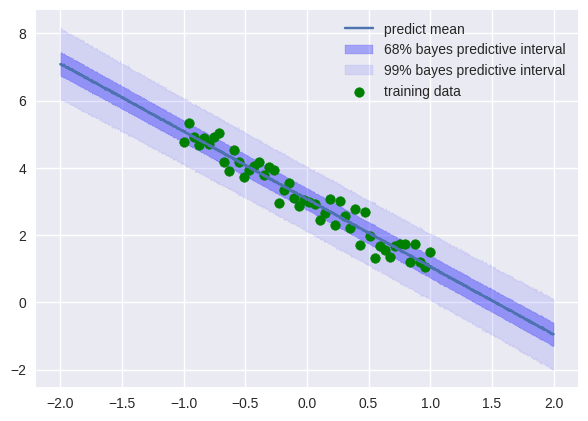## Robust Regression

If data includes outlier, should we exclude that data point? Is there a possibility that outlier inform us important fact, for example that machine is anormaly state? In this section, robust regression model is introduced which can used with data include outliers.

### toy data

def toy_data(a, b, N):
x = np.linspace(-1, 1, N)
y = a * x + b + 0.5*random.normal(random.PRNGKey(0), x.shape)
outlier_index = random.bernoulli(random.PRNGKey(0), 0.1, x.shape)
y += outlier_index*3*random.normal(random.PRNGKey(0), outlier_index.shape)
return x, y

x_data, y_data = toy_data(2, -1, 50)
plt.plot(x_data, y_data, "o")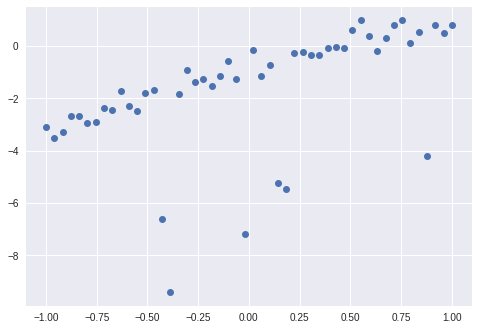### Robust regression model

A robust regression model uses T distribution as likelihood function instead of normal distribution. T distribution have a parameter $\nu$ which determine itselfs form, for example $\nu = 1$, that form is equal to Cauchy distribution, and $\nu \rightarrow \infty$ that asymptotic to normal distributions. Now let's using $\nu = 2$ T distribution.

\begin{align} a &\sim {\rm Normal}(0, 100) \\ b &\sim {\rm Normal}(0, 100) \\ \sigma & \sim {\rm LogNormal}(0, 2) \\ y _ i &\sim {\rm T} _ {2}(ax _ i+b, \sigma) \end{align}

def model(x, y, N=100):
a = sample("a", dist.Normal(0, 100))
b = sample("b", dist.Normal(0, 100))

sigma = sample("sigma", dist.LogNormal(0, 2))

with plate("data", N):
sample("obs", dist.StudentT(2, a*x+b, sigma), obs=y)


### inference and result

kernel = NUTS(model)
mcmc = MCMC(kernel, num_warmup=500, num_samples=2000)
mcmc.run(random.PRNGKey(0), x_data, y_data, x_data.shape)
mcmc.print_summary()

mean       std    median      5.0%     95.0%     n_eff     r_hat
a      2.07      0.11      2.07      1.90      2.25   2012.56      1.00
b     -1.00      0.06     -1.00     -1.10     -0.90   1534.53      1.00
sigma      0.36      0.06      0.36      0.27      0.47   1231.46      1.00

Number of divergences: 0


### predictive

samples = mcmc.get_samples()
predictive = Predictive(model, samples)
index_points = np.linspace(-2., 2., 1500)
predictive_samples = predictive.get_samples(
random.PRNGKey(1),
index_points,
None,
index_points.shape)["obs"]

mean = predictive_samples.mean(0)
std = predictive_samples.std(0)

lower1 = mean - std
upper1 = mean + std
lower3 = mean - 3*std
upper3 = mean + 3*std

plt.figure(figsize=(8, 5), dpi=100)
plt.plot(index_points, mean)
plt.fill_between(index_points.squeeze(), lower1, upper1, alpha=0.3, color="b")
plt.fill_between(index_points.squeeze(), lower3, upper3, alpha=0.1, color="b")
plt.scatter(x_data, y_data, color="g")
plt.legend(["predict mean",
"68% bayes predictive interval",
"99% bayes predictive interval",
"training data"])
plt.ylim([-15, 15])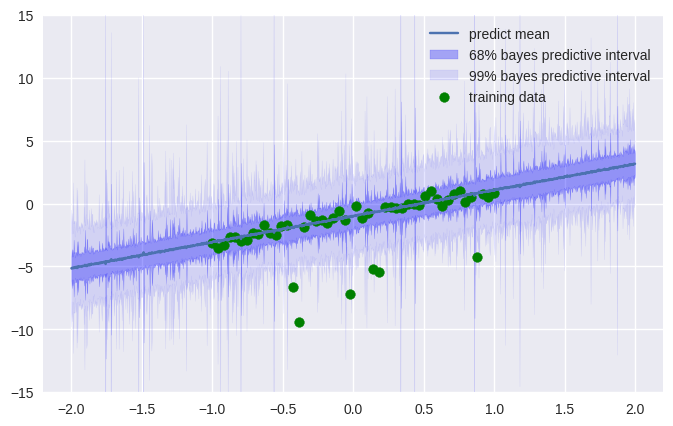That sampling come from T distribution. When we are interested in regression model $y = ax + b$, we maybe want to focus on only a and b random effect. In this case we can use dist.Delta distribution which return directly inputs determistically.

def reg_model(x, N):
a = sample("a", dist.Normal(0, 100))
b = sample("b", dist.Normal(0, 100))

sigma = sample("sigma", dist.LogNormal(0, 2))

with plate("data", N):
sample("obs", dist.Delta(a*x+b))

reg_predictive = Predictive(reg_model, samples)

index_points = np.linspace(-2., 2., 1500)
predictive_samples = reg_predictive.get_samples(
random.PRNGKey(1),
index_points,
index_points.shape)["obs"]

mean = predictive_samples.mean(0)
std = predictive_samples.std(0)

lower1 = mean - std
upper1 = mean + std
lower3 = mean - 3*std
upper3 = mean + 3*std

plt.figure(figsize=(8, 5), dpi=100)
plt.plot(index_points, mean)
plt.fill_between(index_points.squeeze(), lower1, upper1, alpha=0.3, color="b")
plt.fill_between(index_points.squeeze(), lower3, upper3, alpha=0.1, color="b")
plt.scatter(x_data, y_data, color="g")
plt.legend(["predict mean",
"68% bayes predictive interval",
"99% bayes predictive interval",
"training data"])
plt.ylim([-15, 15])